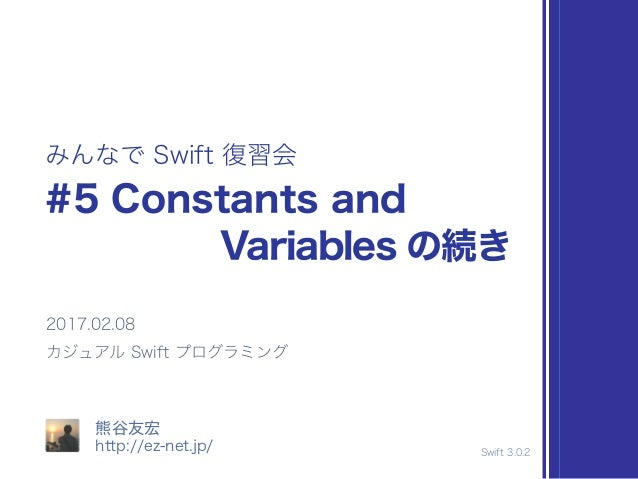Successfully reported this slideshow.Upcoming SlideShare
×

# みんなで Swift 復習会での談笑用スライド – 5th #minna_de_swift

398 views

Published on

みんなで Swift 復習会 (http://cswift.connpass.com) の談笑で使った資料です。これを題材に２時間ばかり、集まったみんなと談笑しました。

Published in: Software
• Full Name
Comment goes here.

Are you sure you want to Yes No• Be the first to comment

### みんなで Swift 復習会での談笑用スライド – 5th #minna_de_swift

1. 1. let π = 3.14 let ＃ = "minnna_de_swift" let 📱 = "iPhone 7" // 使えない文字もある let ⛩ = "伏見稲荷大社"
2. 2. let `repeat` = 10 print(`repeat`)
3. 3. let maxCapacity = 100 var amount = 10 maxCapacity = 20 amount = 20
4. 4. print("A") print("A", "B") print("A", "B", separator: "&") print("A", "B", separator: "&", terminator: “;")
5. 5. let a = 100 let b = 200 // 文字列の連結 print("a = " + String(a) + ", b = " + String(b)) // 文字列補完構文 print("a = (a), b = (b)”)
6. 6. let value = 10 let string = String(describing: value)
7. 7. String(describing: Int.self) // Int String(reflecting: Int.self) // Swift.Int let v = Int.self as Int.Type? print(v) // "Optional(Swift.Int)n"
8. 8. Mirror(reflecting: value) for child in Mirror(reflecting: value).children { print(child) }
9. 9. extension MyData : CustomPlaygroundQuickLookable { var customPlaygroundQuickLook: PlaygroundQuickLook { return .text("My Data") } }